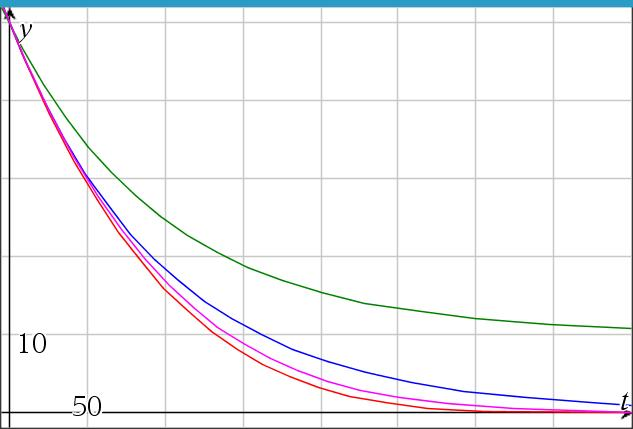# Brine mixture, case 4, inflow≠outflow, brine in

Up a level : Differential Equations
Previous page : Brine mixture, case 3, inflow=outflow, brine in
Next page : Solving the mixture problem using Euler’s step methodCase 4: r1r2, k1>0

This will be a combination of case 2 and 3. We now have the equation$\frac{{dy}}{{dt}} = {k_1}{r_1} - \frac{y}{V}{r_2} = {k_1}{r_1} - \frac{y}{{{V_0} - \left( {{r_2} - {r_1}} \right)t}}{r_2}$

I.e. an equation of the form$\frac{{dy}}{{dx}} = A - \frac{{By}}{{C + Dx}}$

As you might see, if you try, this is not separable. It is a first order linear differential equation, i.e. something that could fit into the pattern$y'{\rm{ }} + {\rm{ }}p\left( x \right)y{\rm{ }} = {\rm{ }}q\left( x \right)$

In this case we have that$y'{\rm{ }} + {\rm{ }}\frac{{{r_2}}}{{{V_0} - \left( {{r_2} - {r_1}} \right)t}}y{\rm{ }} = {\rm{ }}{k_1}{r_1}$

From earlier on we have seen that this can be solved using an integrating factor,$I = {e^{\int {p(t)dt} }}$

This will be rather messy, so we will, for this time, start with an example, and come back to the general case a bit further on.

Example

Say we have Say we have V=V0=2000 l, r1 = 15 l/s, r2 = 20 l/s, k1=10/2000 kg/l and y0 = 50 kg. This would give us the equation$\frac{{dy}}{{dt}} = \frac{{10}}{{2000}} \cdot 15 - \frac{y}{{2000 - \left( {20 - 15} \right)t}}20$

or$\frac{{dy}}{{dt}} = \frac{3}{{40}} - \frac{4}{{400 - t}}y$

and thus$y' + \frac{4}{{400 - t}}y = \frac{3}{{40}}$

The integrating factor will now be$\begin{array}{l} I = {e^{\int {p(t)dt} }} = {e^{\int {\frac{4}{{400 - t}}dt} }} = {e^{ - 4\ln \left( {400 - t} \right)}}\\ \;\; = {e^{\ln {{\left( {400 - t} \right)}^{ - 4}}}} = {\left( {400 - t} \right)^{ - 4}} \end{array}$

This will give us that$Iy' + I\frac{4}{{400 - t}}y = \frac{3}{{40}}I$

will become${\left( {400 - t} \right)^{ - 4}}y' + {\left( {400 - t} \right)^{ - 4}}\frac{4}{{400 - t}}y = - \frac{3}{{40}}{\left( {400 - t} \right)^{ - 4}}$

or${\left( {400 - t} \right)^{ - 4}}y' + 4{\left( {400 - t} \right)^{ - 5}}y = \frac{3}{{40}}{\left( {400 - t} \right)^{ - 4}}$

This can now be rewritten as${\left( {4{{\left( {400 - t} \right)}^{ - 4}}y} \right)^\prime } = \frac{3}{{40}}{\left( {400 - t} \right)^{ - 4}}$

Integrating both sides with respect to t will give us$4{\left( {400 - t} \right)^{ - 4}}y = \frac{3}{{40}}\int {{{\left( {400 - t} \right)}^{ - 4}}dt = } - \frac{3}{{40}}\frac{{{{\left( {400 - t} \right)}^{ - 3}}}}{{ - 3}} + {C_1}$

or,$y = \frac{1}{{160}}\left( {400 - t} \right) + {C_2}{\left( {400 - t} \right)^4}$

As before we can factorize out the 400 to get$y = \frac{{400}}{{160}}\left( {1 - \frac{t}{{400}}} \right) + C \cdot {\left( {1 - \frac{t}{{400}}} \right)^4} = C \cdot {\left( {1 - \frac{t}{{400}}} \right)^4} + \frac{5}{2}\left( {1 - \frac{t}{{400}}} \right)$

To find C:$50 = C + \frac{5}{2} \Rightarrow C = \frac{{95}}{2}$

This will finally give us$y = \frac{{95}}{2}{\left( {1 - \frac{t}{{400}}} \right)^4} + \frac{5}{2}\left( {1 - \frac{t}{{400}}} \right)$

This would be the second to lowest curve in the graph below.Back to the general case

It will be easier to work with$y' + \frac{{By}}{{C + Dt}} = A$

than$y'{\rm{ }} + {\rm{ }}\frac{{{r_2}}}{{{V_0} - \left( {{r_2} - {r_1}} \right)t}}y{\rm{ }} = {\rm{ }}{k_1}{r_1}$

We have$\int {p(t)dt} = \int {\frac{B}{{C + Dt}}dt} = \frac{B}{D}\ln \left( {C + Dt} \right)$

or$\int {p(t)dt} = \ln {\left( {C + Dt} \right)^{B/D}}$

This gives us$I = {e^{\int {p(t)dt} }} = {\left( {C + Dt} \right)^{B/D}}$

and$Iy' + I\frac{{By}}{{C + Dt}} = IA$

or${\left( {C + Dt} \right)^{B/D}}y' + {\left( {C + Dt} \right)^{B/D}}\frac{{By}}{{C + Dt}} = {\left( {C + Dt} \right)^{B/D}}A$

and thus${\left( {C + Dt} \right)^{B/D}}y' + {\left( {C + Dt} \right)^{B/D - 1}}By = {\left( {C + Dt} \right)^{B/D}}A$

that can be written as${\left( {{{\left( {C + Dt} \right)}^{B/D}}y} \right)^\prime } = {\left( {C + Dt} \right)^{B/D}}A$

Integrating this gives us${\left( {C + Dt} \right)^{B/D}}y = A\int {{{\left( {C + Dt} \right)}^{B/D}}dt = \frac{A}{D}\frac{{{{\left( {C + Dt} \right)}^{B/D + 1}}}}{{B/D + 1}}} + {K_1}$

where K1 is the constant of integration.

This gives us$y = A\frac{{\left( {C + Dt} \right)}}{{B + D}} + {K_1}{\left( {C + Dt} \right)^{ - B/D}}$

Filling in what we had initially we will get$y = {k_1}{r_1}\frac{{\left( {{V_0} - \left( {{r_2} - {r_1}} \right)t} \right)}}{{{r_2} - \left( {{r_2} - {r_1}} \right)}} + {K_1}{\left( {{V_0} - \left( {{r_2} - {r_1}} \right)t} \right)^{\frac{{{r_2}}}{{{r_2} - {r_1}}}}}$

or$y = {V_0}{k_1}\left( {1 - \frac{{{r_2} - {r_1}}}{{{V_0}}}t} \right) + K{\left( {1 - \frac{{{r_2} - {r_1}}}{{{V_0}}}t} \right)^{\frac{{{r_2}}}{{{r_2} - {r_1}}}}}$

Here we did the same trick as before, pulling out factors of V0, and then let it be absorbed by the constant of integration.

Finally, we find K:${y_0} = {V_0}{k_1} + K \Rightarrow K = {y_0} - {V_0}{k_1}$

This will give us:$y = \left( {{y_0} - {V_0}{k_1}} \right){\left( {1 - \frac{{{r_2} - {r_1}}}{{{V_0}}}t} \right)^{\frac{{{r_2}}}{{{r_2} - {r_1}}}}} + {V_0}{k_1}\left( {1 - \frac{{{r_2} - {r_1}}}{{{V_0}}}t} \right)$

We can see that case 2 is just a special case of case 4.

When general is not general

This is an example of when the “general” solution will not cover all cases. If one would have started with the “general” case, one would end up with the solution above, but as one can see, this cannot be the general solution. Why not?  Because we need to divide by r2r1, and that would be 0 if r2=r1, we must thus examine that case too, and that would lead us to the cases 1 and 2.Up a level : Differential Equations
Previous page : Brine mixture, case 3, inflow=outflow, brine in
Next page : Solving the mixture problem using Euler’s step methodLast modified: Dec 28, 2020 @ 16:44